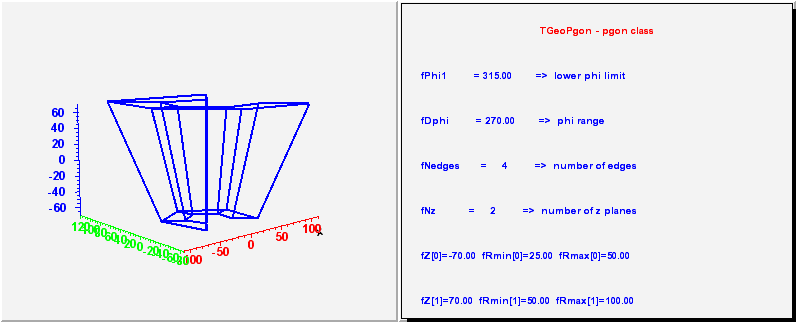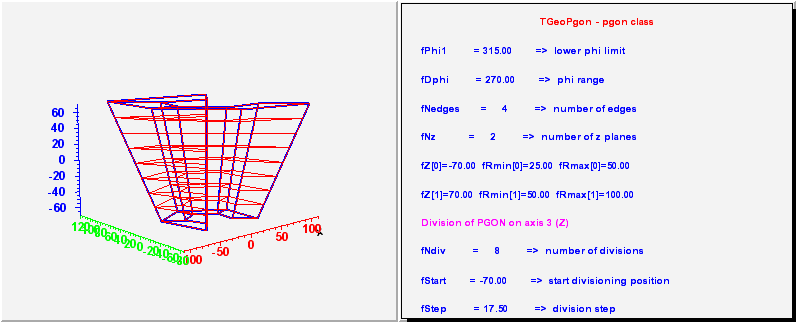library: libGeom#include "TGeoPgon.h"

# TGeoPgon

class description - source file - inheritance tree (.pdf)

## class TGeoPgon : public TGeoPcon

Inheritance Chart:
 TObject
<-
TNamed
<-
TGeoShape
<-
TGeoBBox
<-
TGeoPcon
<-
TGeoPgon
```
protected:
Int_t GetPhiCrossList(Double_t* point, Double_t* dir, Int_t istart, Double_t* sphi, Int_t* iphi, Double_t stepmax = TGeoShape::Big()) const
Bool_t IsCrossingSlice(Double_t* point, Double_t* dir, Int_t iphi, Double_t sstart, Int_t& ipl, Double_t& snext, Double_t stepmax) const
void LocatePhi(Double_t* point, Int_t& ipsec) const
Double_t Rpg(Double_t z, Int_t ipl, Bool_t inner, Double_t& a, Double_t& b) const
Double_t Rproj(Double_t z, Double_t* point, Double_t* dir, Double_t cphi, Double_t sphi, Double_t& a, Double_t& b) const
Bool_t SliceCrossing(Double_t* point, Double_t* dir, Int_t nphi, Int_t* iphi, Double_t* sphi, Double_t& snext, Double_t stepmax) const
Bool_t SliceCrossingIn(Double_t* point, Double_t* dir, Int_t nphi, Int_t* iphi, Double_t* sphi, Double_t& snext, Double_t stepmax) const
Bool_t SliceCrossingInZ(Double_t* point, Double_t* dir, Int_t nphi, Int_t* iphi, Double_t* sphi, Double_t& snext, Double_t stepmax) const
Bool_t SliceCrossingZ(Double_t* point, Double_t* dir, Int_t nphi, Int_t* iphi, Double_t* sphi, Double_t& snext, Double_t stepmax) const

public:
TGeoPgon()
TGeoPgon(Double_t phi, Double_t dphi, Int_t nedges, Int_t nz)
TGeoPgon(const char* name, Double_t phi, Double_t dphi, Int_t nedges, Int_t nz)
TGeoPgon(Double_t* params)
TGeoPgon(const TGeoPgon&)
virtual ~TGeoPgon()
static TClass* Class()
virtual void ComputeBBox()
virtual void ComputeNormal(Double_t* point, Double_t* dir, Double_t* norm)
virtual Bool_t Contains(Double_t* point) const
virtual void DefineSection(Int_t snum, Double_t z, Double_t rmin, Double_t rmax)
virtual Int_t DistancetoPrimitive(Int_t px, Int_t py)
virtual Double_t DistFromInside(Double_t* point, Double_t* dir, Int_t iact = 1, Double_t step = TGeoShape::Big(), Double_t* safe = 0) const
virtual Double_t DistFromOutside(Double_t* point, Double_t* dir, Int_t iact = 1, Double_t step = TGeoShape::Big(), Double_t* safe = 0) const
virtual TGeoVolume* Divide(TGeoVolume* voldiv, const char* divname, Int_t iaxis, Int_t ndiv, Double_t start, Double_t step)
virtual void GetBoundingCylinder(Double_t* param) const
virtual Int_t GetByteCount() const
virtual TGeoShape* GetMakeRuntimeShape(TGeoShape*, TGeoMatrix*) const
Int_t GetNedges() const
virtual Int_t GetNmeshVertices() const
virtual Int_t GetNsegments() const
virtual void InspectShape() const
virtual TClass* IsA() const
virtual TBuffer3D* MakeBuffer3D() const
TGeoPgon& operator=(const TGeoPgon&)
virtual void Paint(Option_t* option)
virtual Double_t Safety(Double_t* point, Bool_t in = kTRUE) const
Double_t SafetyToSegment(Double_t* point, Int_t ipl, Int_t iphi, Bool_t in, Double_t safphi, Double_t safmin = TGeoShape::Big()) const
virtual void SetDimensions(Double_t* param)
virtual void SetPoints(Double_t* buff) const
virtual void SetPoints(Float_t* buff) const
virtual void SetSegsAndPols(TBuffer3D* buff) const
virtual void ShowMembers(TMemberInspector& insp, char* parent)
virtual void Sizeof3D() const
virtual void Streamer(TBuffer& b)
void StreamerNVirtual(TBuffer& b)
```

### Data Members

```
protected:
Int_t fNedges  number of edges (at least one)
```

## Class Description

``` TGeoPgon - a polygone. It has at least 10 parameters :
- the lower phi limit;
- the range in phi;
- the number of edges on each z plane;
- the number of z planes (at least two) where the inner/outer
- z coordinate, inner and outer radius for each z plane

_____________________________________________________________________________
```
/**/

/**/

TGeoPgon()
``` dummy ctor
```

TGeoPgon(Double_t phi, Double_t dphi, Int_t nedges, Int_t nz) :TGeoPcon(phi, dphi, nz)
``` Default constructor
```

TGeoPgon(const char *name, Double_t phi, Double_t dphi, Int_t nedges, Int_t nz) :TGeoPcon(name, phi, dphi, nz)
``` Default constructor
```

TGeoPgon(Double_t *param) :TGeoPcon()
``` Default constructor in GEANT3 style
param = phi1
param = dphi
param = nedges
param = nz

param = z1
param = Rmin1
param = Rmax1
...
```

~TGeoPgon()
``` destructor
```

void ComputeBBox()
``` compute bounding box for a polygone
```

void ComputeNormal(Double_t *point, Double_t *dir, Double_t *norm)
``` Compute normal to closest surface from POINT.
```

Bool_t Contains(Double_t *point) const
``` test if point is inside this shape
check total z range
```

void DefineSection(Int_t snum, Double_t z, Double_t rmin, Double_t rmax)
``` defines z position of a section plane, rmin and rmax at this z.
```

Double_t DistFromInside(Double_t *point, Double_t *dir, Int_t iact, Double_t step, Double_t *safe) const
``` compute distance from inside point to surface of the polygone
first find out in which Z section the point is in
```

void LocatePhi(Double_t *point, Int_t &ipsec) const

Int_t GetPhiCrossList(Double_t *point, Double_t *dir, Int_t istart, Double_t *sphi, Int_t *iphi, Double_t stepmax) const
```printf("   PHI crossing list:\n");
```

Bool_t SliceCrossingInZ(Double_t *point, Double_t *dir, Int_t nphi, Int_t *iphi, Double_t *stepphi, Double_t &snext, Double_t stepmax) const

Bool_t SliceCrossingZ(Double_t *point, Double_t *dir, Int_t nphi, Int_t *iphi, Double_t *stepphi, Double_t &snext, Double_t stepmax) const

Bool_t SliceCrossingIn(Double_t *point, Double_t *dir, Int_t nphi, Int_t *iphi, Double_t *stepphi, Double_t &snext, Double_t stepmax) const
``` Check boundary crossing inside phi slices. Return distance snext to first crossing
if smaller than stepmax.
printf("   SliceCrossingIn. stepmax = %f\n", stepmax);
```

Bool_t SliceCrossing(Double_t *point, Double_t *dir, Int_t nphi, Int_t *iphi, Double_t *stepphi, Double_t &snext, Double_t stepmax) const
``` Check boundary crossing inside phi slices. Return distance snext to first crossing
if smaller than stepmax.
```

Bool_t IsCrossingSlice(Double_t *point, Double_t *dir, Int_t iphi, Double_t sstart, Int_t &ipl, Double_t &snext, Double_t stepmax) const
``` Check crossing of a given pgon slice, from a starting point inside the slice
```

Double_t DistFromOutside(Double_t *point, Double_t *dir, Int_t iact, Double_t step, Double_t *safe) const
``` compute distance from outside point to surface of the polygone
```

Int_t DistancetoPrimitive(Int_t px, Int_t py)
``` compute closest distance from point px,py to each corner
```

TGeoVolume* Divide(TGeoVolume *voldiv, const char *divname, Int_t iaxis, Int_t ndiv, Double_t start, Double_t step)
```--- Divide this polygone shape belonging to volume "voldiv" into ndiv volumes
called divname, from start position with the given step. Returns pointer
to created division cell volume in case of Z divisions. Phi divisions are
allowed only if nedges%ndiv=0 and create polygone "segments" with nedges/ndiv edges.
Z divisions can be performed if the divided range is in between two consecutive Z planes.
In case a wrong division axis is supplied, returns pointer to volume that was divided.
```

void GetBoundingCylinder(Double_t *param) const
```--- Fill vector param with the bounding cylinder parameters. The order
is the following : Rmin, Rmax, Phi1, Phi2
```

void InspectShape() const

TBuffer3D* MakeBuffer3D() const
``` Creates a TBuffer3D describing *this* shape.
Coordinates are in local reference frame.
```

void Paint(Option_t *option)
``` Paint this shape according to option
```

void SetSegsAndPols(TBuffer3D *buff) const
``` Fill TBuffer3D structure for segments and polygons.
```

Double_t Rpg(Double_t z, Int_t ipl, Bool_t inner, Double_t &a, Double_t &b) const
``` Computes projected pgon radius (inner or outer) corresponding to a given Z
value. Fills corresponding coefficients of:
Rpg(z) = a + b*z
Note: ipl must be in range [0,fNz-2]
```

Double_t Rproj(Double_t z, Double_t *point, Double_t *dir, Double_t cphi, Double_t sphi, Double_t &a, Double_t &b) const
``` Computes projected distance at a given Z for a given ray inside a given sector
and fills coefficients:
Rproj = a + b*z
```

Double_t SafetyToSegment(Double_t *point, Int_t ipl, Int_t iphi, Bool_t in, Double_t safphi, Double_t safmin) const
``` Compute safety from POINT to segment between planes ipl, ipl+1 within safmin.
```

Double_t Safety(Double_t *point, Bool_t in) const
``` computes the closest distance from given point to this shape, according
to option. The matching point on the shape is stored in spoint.
```

void SetDimensions(Double_t *param)

void SetPoints(Double_t *buff) const
``` create polygone mesh points
```

void SetPoints(Float_t *buff) const
``` create polygone mesh points
```

Int_t GetNmeshVertices() const
``` Return number of vertices of the mesh representation
```

void Sizeof3D() const
```/// fill size of this 3-D object
/    TVirtualGeoPainter *painter = gGeoManager->GetGeomPainter();
/    if (!painter) return;
/    Int_t n;

/    n = fNedges+1;

/    Int_t numPoints = fNz*2*n;
/    Int_t numSegs   = 4*(fNz*n-1+(fDphi == 360));
/    Int_t numPolys  = 2*(fNz*n-1+(fDphi == 360));
```

### Inline Functions

```              Int_t GetByteCount() const
TGeoShape* GetMakeRuntimeShape(TGeoShape*, TGeoMatrix*) const
Int_t GetNedges() const
Int_t GetNsegments() const
TClass* Class()
TClass* IsA() const
void ShowMembers(TMemberInspector& insp, char* parent)
void Streamer(TBuffer& b)
void StreamerNVirtual(TBuffer& b)
TGeoPgon TGeoPgon(const TGeoPgon&)
TGeoPgon& operator=(const TGeoPgon&)
```

Author: Andrei Gheata 31/01/02
Last update: root/geom:\$Name: \$:\$Id: TGeoPgon.cxx,v 1.44 2004/12/07 14:24:57 brun Exp \$